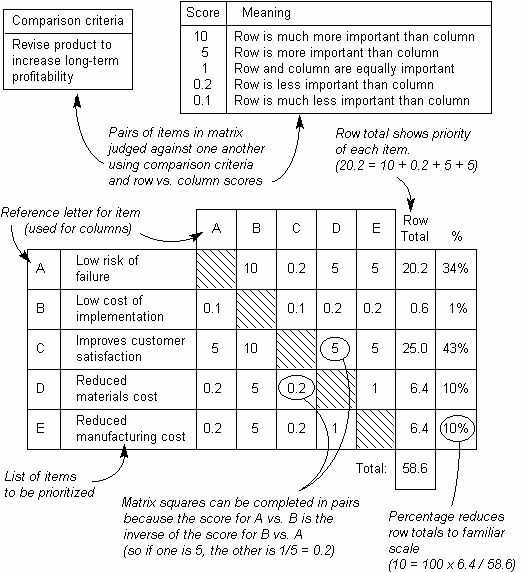The Psychology of Quality and More

Prioritization Matrix: Practical variations

The Quality Toolbook > Prioritization Matrix > Practical variations

Practical variations

• In the Full Analytical Criteria Method, the allocation of weighting and scoring numbers is done through comparing each item against all others by using a standard scoring scheme. This requires a matrix to be calculated for both (a) allocating weights for criteria against the objective, as in step 5, and (b) comparing the list of items against each criterion, as in step 8. Thus, with three criteria, four matrices must be used (one for weighting the criteria and one for scoring each list against the criteria). The detail in this method makes it useful for critical applications, but it is probably too time-consuming for most normal situations.
• The process is described below (and illustrated in Fig. 1):

1. Draw a square matrix, with items both in rows down the left and also in columns along the top.
2. In each square, compare the row item against the column item, using the following scoring scheme:
3. 10 = Row item is much more important than column item

5 = Row item is more important than column item

1 = Row item is equally important to column item

0.2 = Row item is less important than column item

0.1 = Row item is much less important than column item

Squares may be done in diagonally opposite pairs, as if A versus B scores 5 then B versus A must score 0.2. Note that as with other scoring schemes, use of real data will give much better results than subjective guesses.

4. Add up the numbers in each row and convert to a percentage by dividing each one by the total of all row totals.

This final figure is now used for the weight (when comparing criteria against the basic objective) or score (when comparing all items against one criterion).Fig. 1. Full Analytic Criteria Method

• Paired Comparison provides a simple way of prioritizing a list of items when there is a single ranking criterion, as in the illustration below. As each pairwise comparison selects the more important item in the pair rather than giving a score, the result is a prioritized list, but without relative priority shown in a score figure.• Another variation of paired comparison is the Bubble Sort. This is a quick and easy method which can be performed as follows:

1. Write each item to be prioritized on a 3" x 5" card, then place the cards one above another in a vertical column.
2. Compare the top two cards to determine which is the most important. If the lower card is more important, then exchange the positions of the cards. This will result in the more important card being positioned above the less important card.
3. Repeat this pairwise comparison and exchange for the second and third card, then third and fourth card and so on until the bottom of the column is reached.
4. If any cards have been moved during steps (b) and (c), repeat the process for the whole column, starting again with the top two cards, as in step (b).
5. Keep repeating the overall process until no cards are exchanged during a complete pass through the column. The cards are now in priority order.

You can buy books here

And the big
paperback book